Скачать презентацию DATA MINING CLUSTERING Clustering 4 Clustering —

8463b9a356126e8f67312cddd7dcef2c.ppt

• Количество слайдов: 28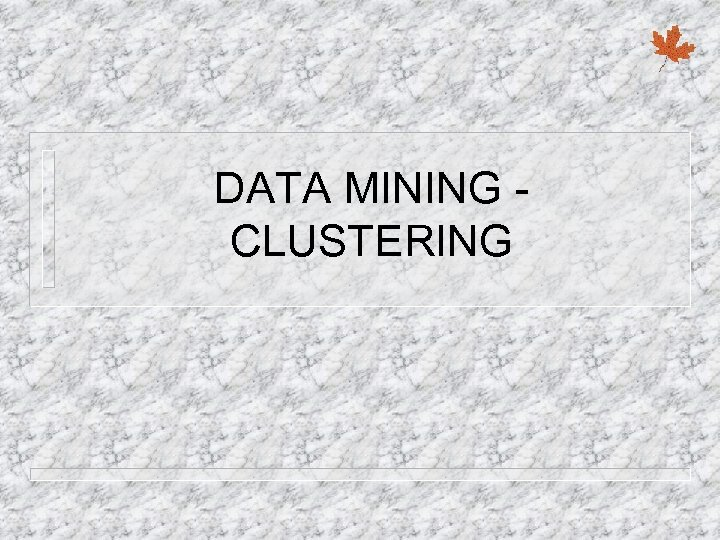DATA MINING CLUSTERING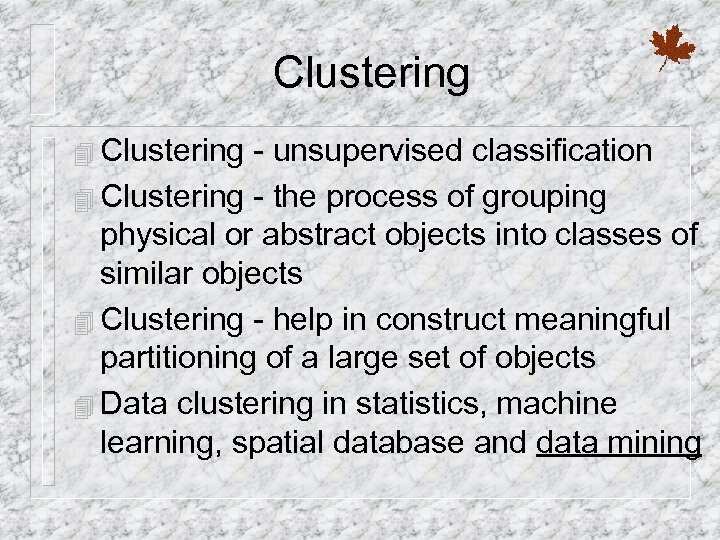Clustering 4 Clustering - unsupervised classification 4 Clustering - the process of grouping physical or abstract objects into classes of similar objects 4 Clustering - help in construct meaningful partitioning of a large set of objects 4 Data clustering in statistics, machine learning, spatial database and data mining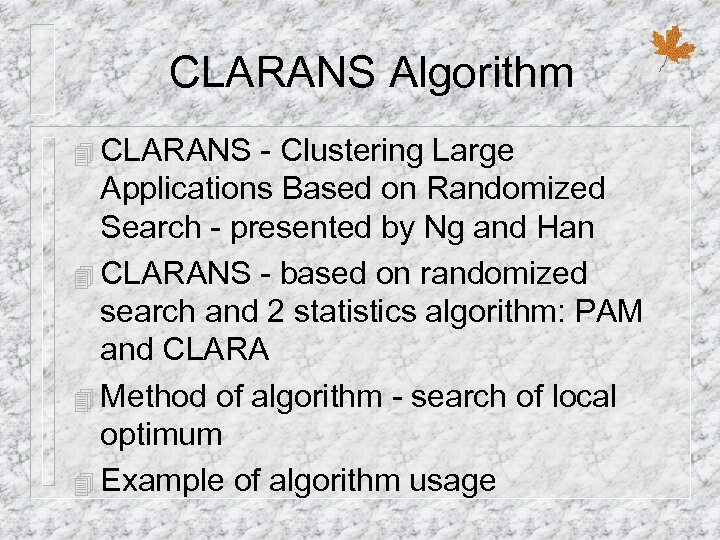CLARANS Algorithm 4 CLARANS - Clustering Large Applications Based on Randomized Search - presented by Ng and Han 4 CLARANS - based on randomized search and 2 statistics algorithm: PAM and CLARA 4 Method of algorithm - search of local optimum 4 Example of algorithm usage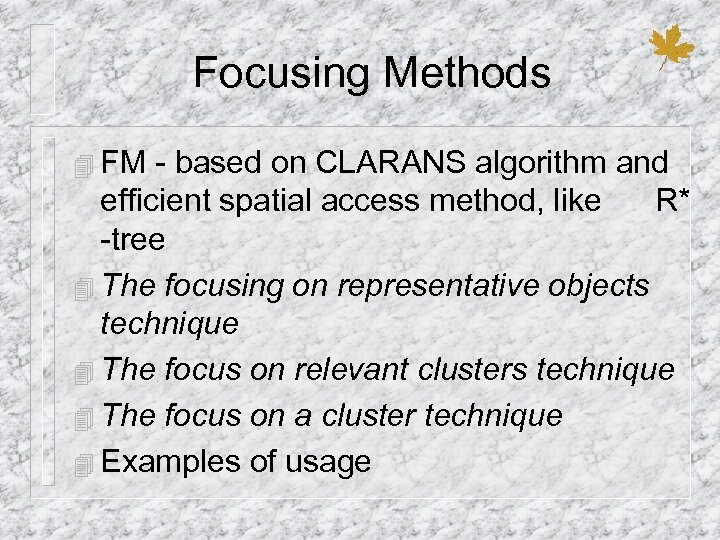Focusing Methods 4 FM - based on CLARANS algorithm and efficient spatial access method, like R* -tree 4 The focusing on representative objects technique 4 The focus on relevant clusters technique 4 The focus on a cluster technique 4 Examples of usage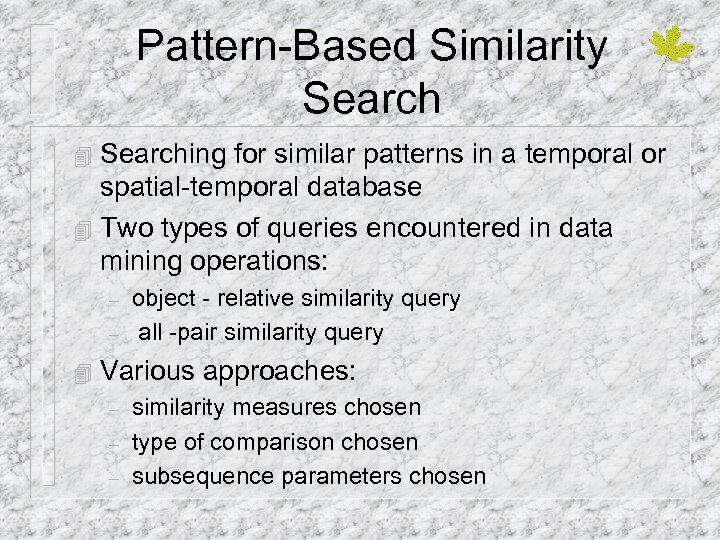Pattern-Based Similarity Searching for similar patterns in a temporal or spatial-temporal database 4 Two types of queries encountered in data mining operations: 4 – – 4 object - relative similarity query all -pair similarity query Various approaches: – – – similarity measures chosen type of comparison chosen subsequence parameters chosen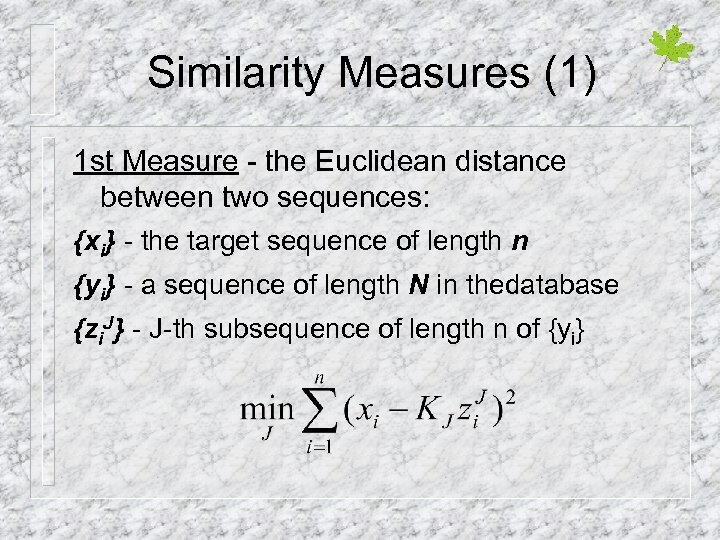Similarity Measures (1) 1 st Measure - the Euclidean distance between two sequences: {xi} - the target sequence of length n {yi} - a sequence of length N in thedatabase {zi. J} - J-th subsequence of length n of {yi}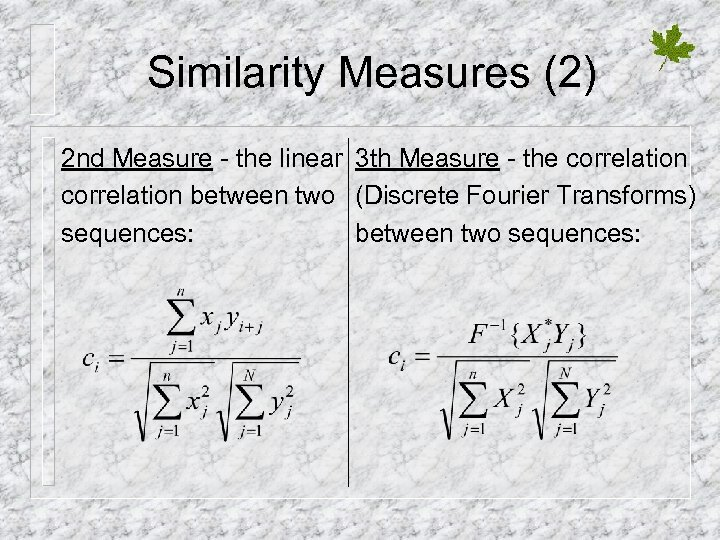Similarity Measures (2) 2 nd Measure - the linear 3 th Measure - the correlation between two (Discrete Fourier Transforms) sequences: between two sequences: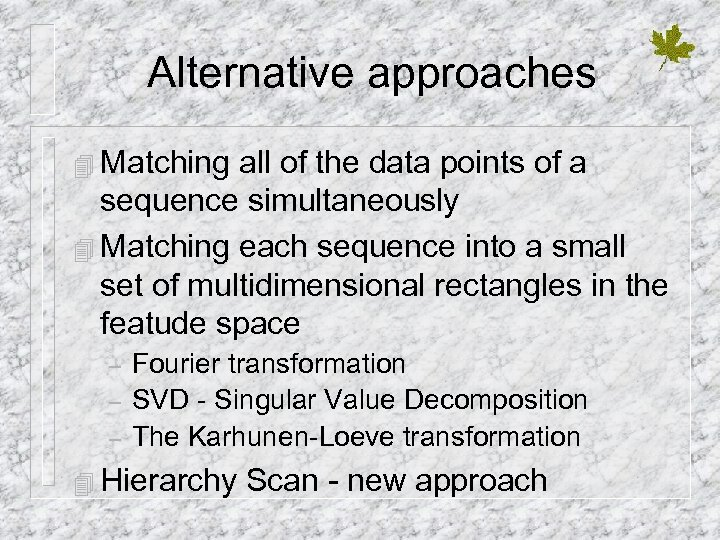Alternative approaches 4 Matching all of the data points of a sequence simultaneously 4 Matching each sequence into a small set of multidimensional rectangles in the featude space – – – Fourier transformation SVD - Singular Value Decomposition The Karhunen-Loeve transformation 4 Hierarchy Scan - new approach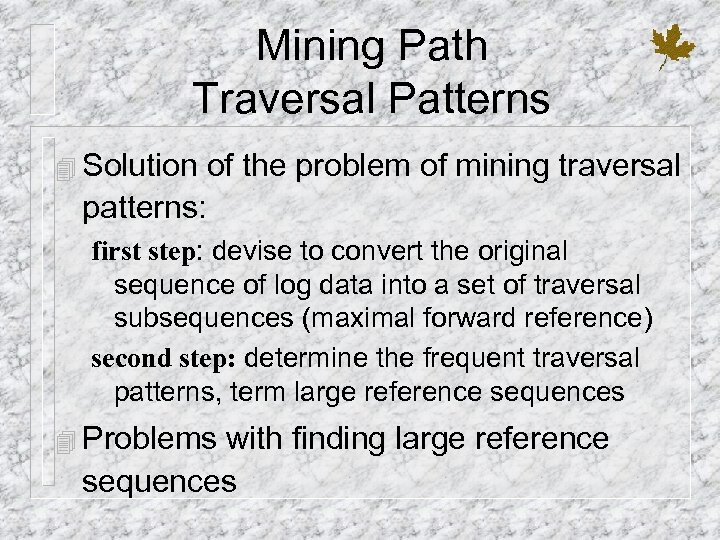Mining Path Traversal Patterns 4 Solution of the problem of mining traversal patterns: first step: devise to convert the original sequence of log data into a set of traversal subsequences (maximal forward reference) second step: determine the frequent traversal patterns, term large reference sequences 4 Problems with finding large reference sequences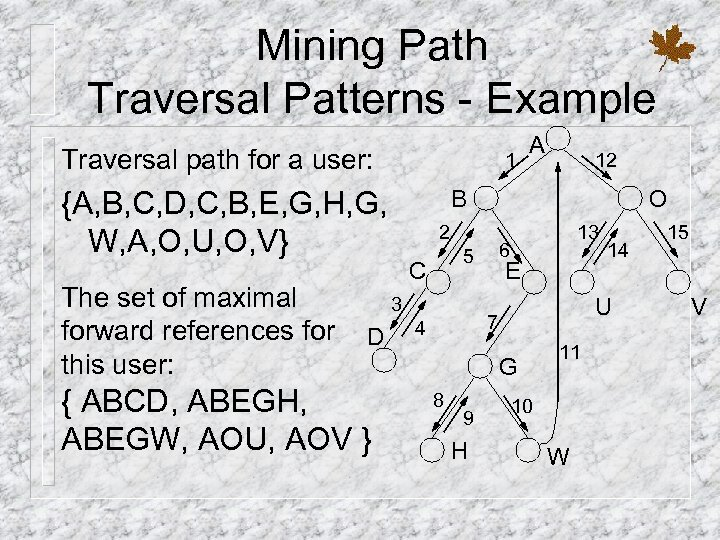Mining Path Traversal Patterns - Example Traversal path for a user: 1 {A, B, C, D, C, B, E, G, H, G, W, A, O, U, O, V} The set of maximal forward references for this user: { ABCD, ABEGH, ABEGW, AOU, AOV } 12 B O 2 C 13 6 5 3 D A E U 7 4 G 8 9 H 14 11 10 W 15 V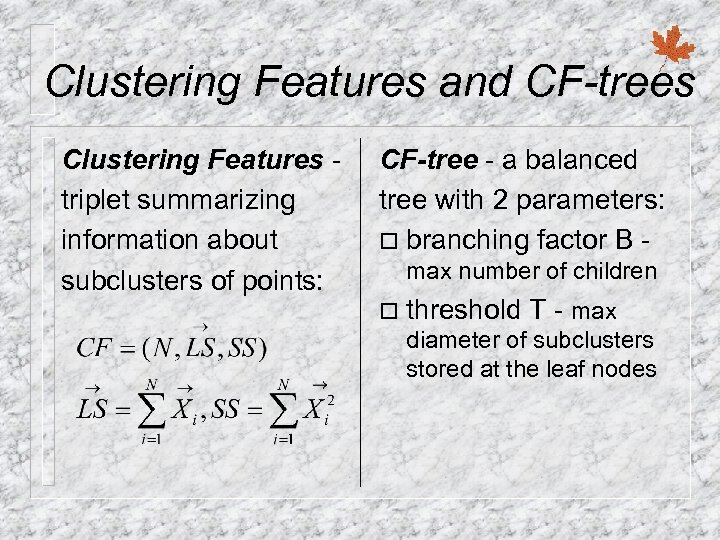Clustering Features and CF-trees Clustering Features triplet summarizing information about subclusters of points: CF-tree - a balanced tree with 2 parameters: ¨ branching factor B max number of children ¨ threshold T - max diameter of subclusters stored at the leaf nodes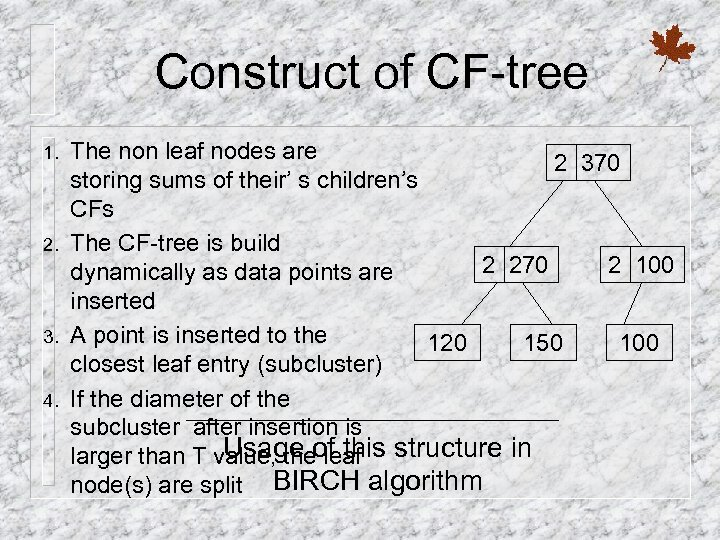Construct of CF-tree 1. 2. 3. 4. The non leaf nodes are 2 370 storing sums of their’ s children’s CFs The CF-tree is build 2 270 2 100 dynamically as data points are inserted A point is inserted to the 120 150 100 closest leaf entry (subcluster) If the diameter of the subcluster after insertion is Usage of this larger than T value, the leaf structure in node(s) are split BIRCH algorithm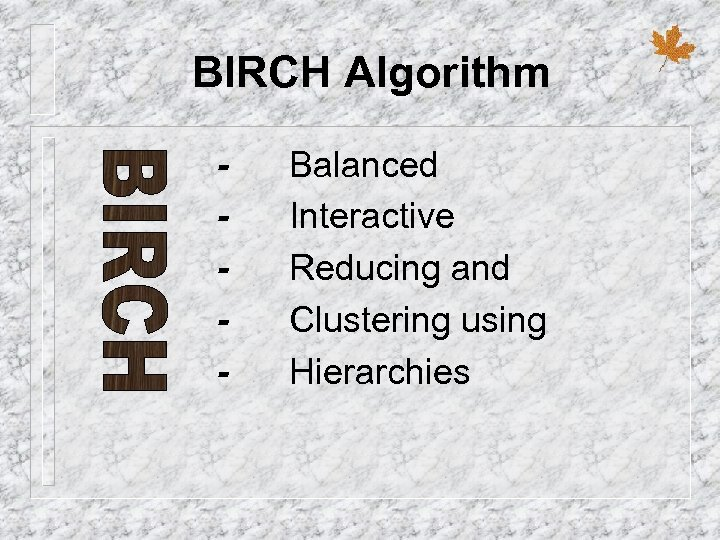BIRCH Algorithm - Balanced Interactive Reducing and Clustering using Hierarchies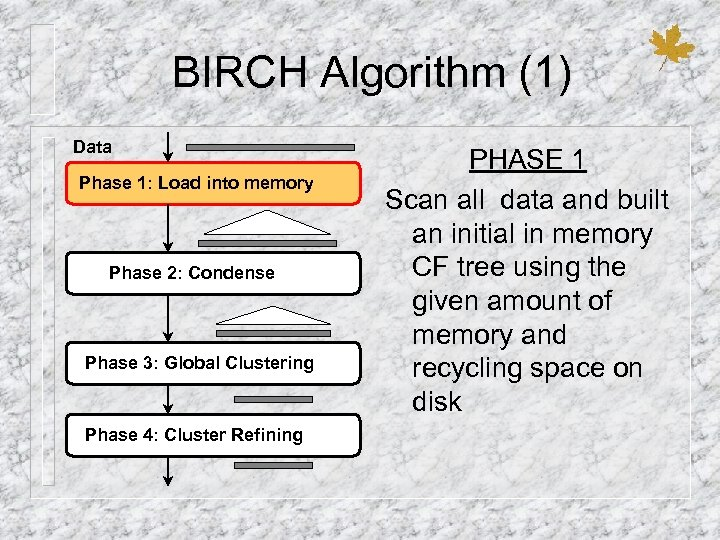BIRCH Algorithm (1) Data Phase 1: Load into memory Phase 2: Condense Phase 3: Global Clustering Phase 4: Cluster Refining PHASE 1 Scan all data and built an initial in memory CF tree using the given amount of memory and recycling space on disk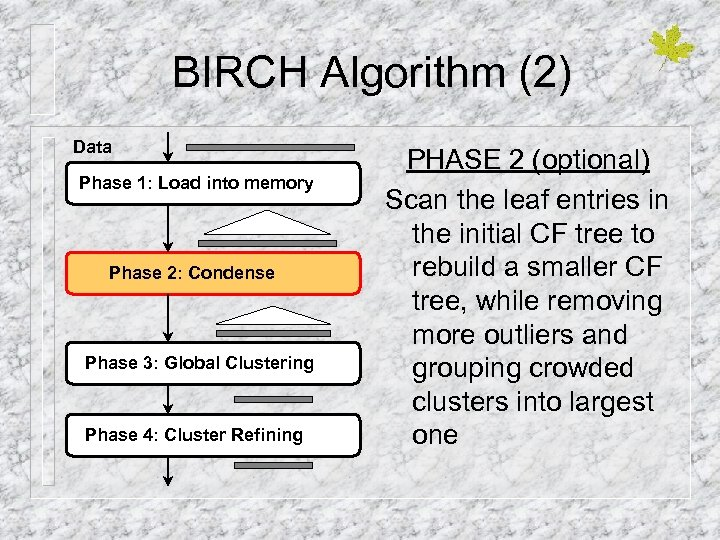BIRCH Algorithm (2) Data Phase 1: Load into memory Phase 2: Condense Phase 3: Global Clustering Phase 4: Cluster Refining PHASE 2 (optional) Scan the leaf entries in the initial CF tree to rebuild a smaller CF tree, while removing more outliers and grouping crowded clusters into largest one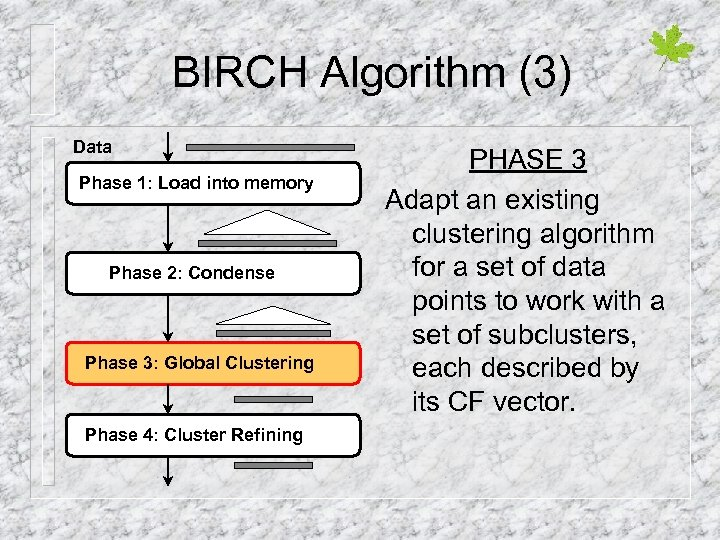BIRCH Algorithm (3) Data Phase 1: Load into memory Phase 2: Condense Phase 3: Global Clustering Phase 4: Cluster Refining PHASE 3 Adapt an existing clustering algorithm for a set of data points to work with a set of subclusters, each described by its CF vector.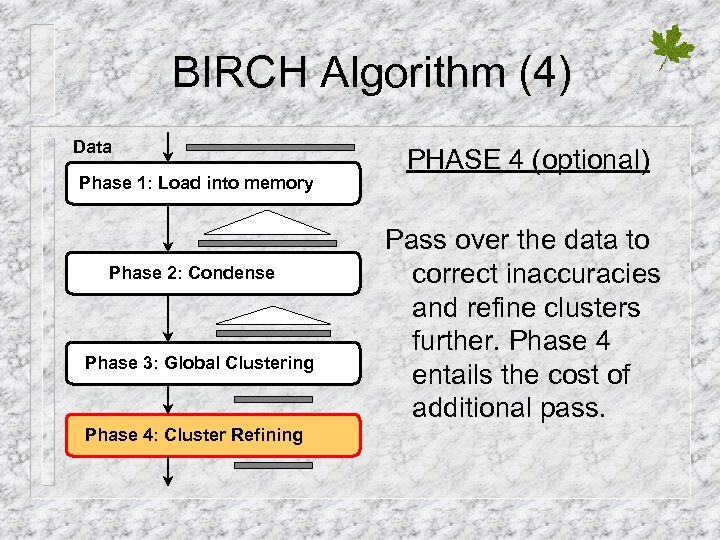BIRCH Algorithm (4) Data Phase 1: Load into memory Phase 2: Condense Phase 3: Global Clustering Phase 4: Cluster Refining PHASE 4 (optional) Pass over the data to correct inaccuracies and refine clusters further. Phase 4 entails the cost of additional pass.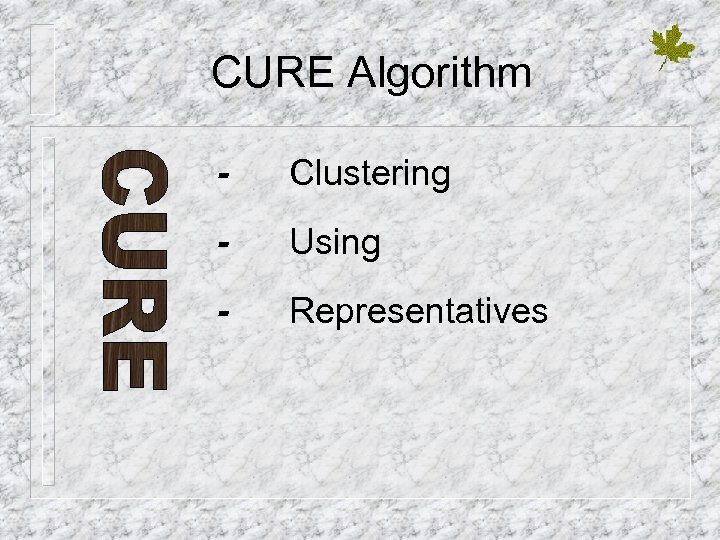CURE Algorithm - Clustering - Using - Representatives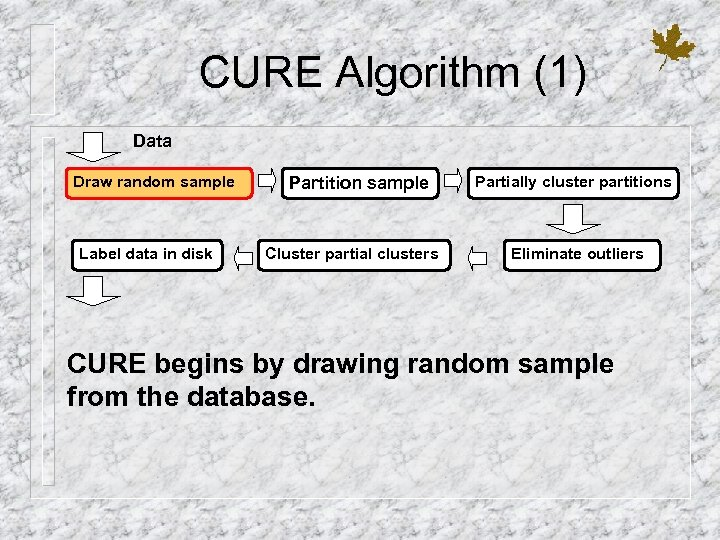CURE Algorithm (1) Data Draw random sample Label data in disk Partition sample Cluster partial clusters Partially cluster partitions Eliminate outliers CURE begins by drawing random sample from the database.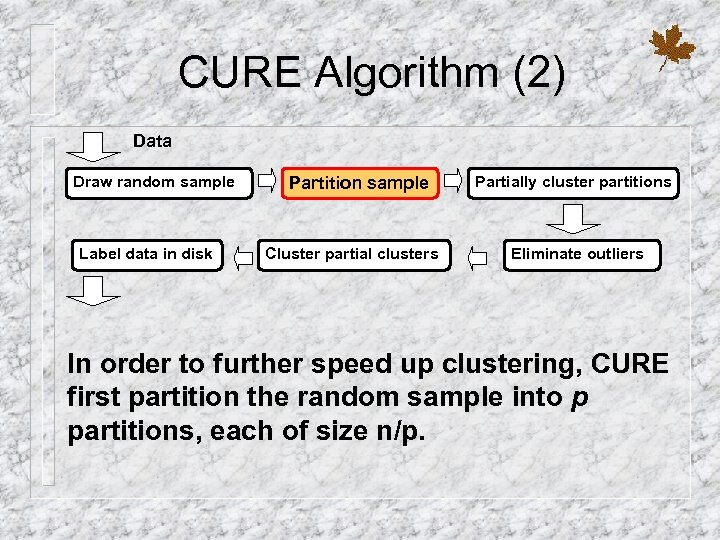CURE Algorithm (2) Data Draw random sample Label data in disk Partition sample Cluster partial clusters Partially cluster partitions Eliminate outliers In order to further speed up clustering, CURE first partition the random sample into p partitions, each of size n/p.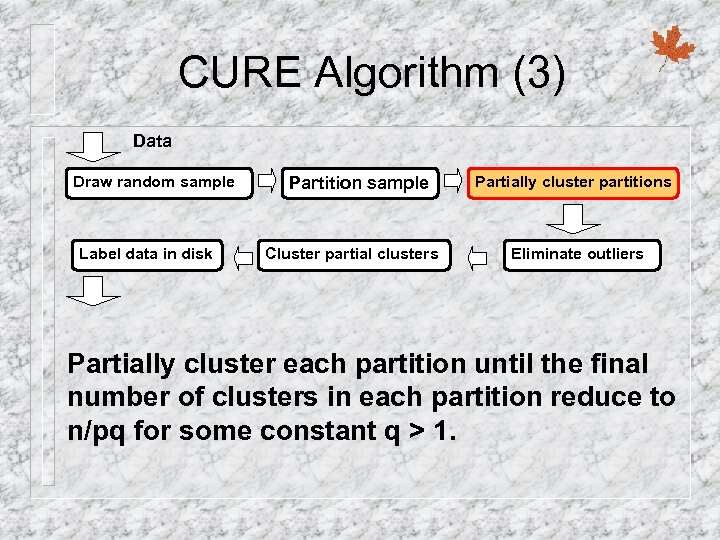CURE Algorithm (3) Data Draw random sample Label data in disk Partition sample Cluster partial clusters Partially cluster partitions Eliminate outliers Partially cluster each partition until the final number of clusters in each partition reduce to n/pq for some constant q > 1.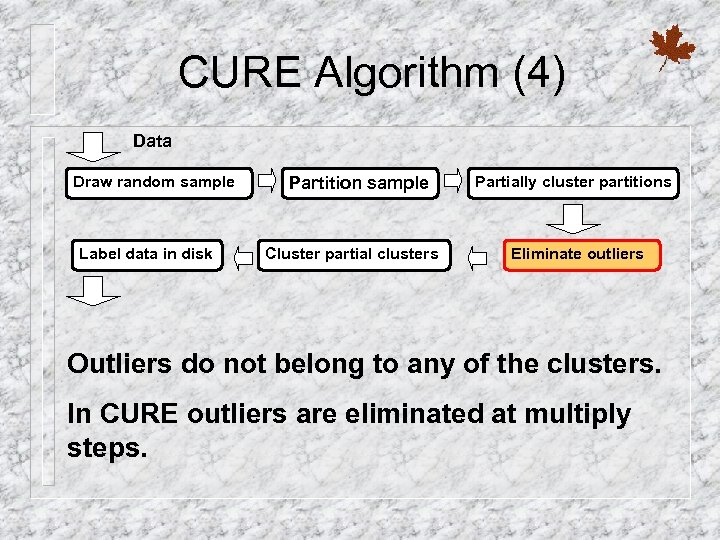CURE Algorithm (4) Data Draw random sample Label data in disk Partition sample Cluster partial clusters Partially cluster partitions Eliminate outliers Outliers do not belong to any of the clusters. In CURE outliers are eliminated at multiply steps.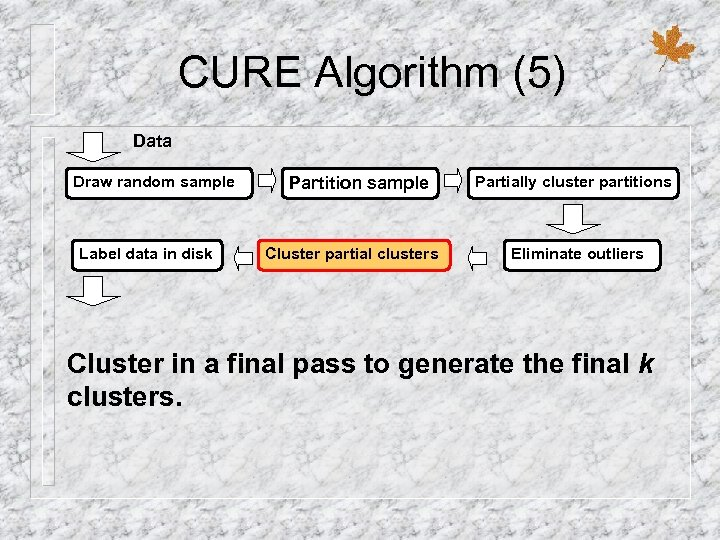CURE Algorithm (5) Data Draw random sample Label data in disk Partition sample Cluster partial clusters Partially cluster partitions Eliminate outliers Cluster in a final pass to generate the final k clusters.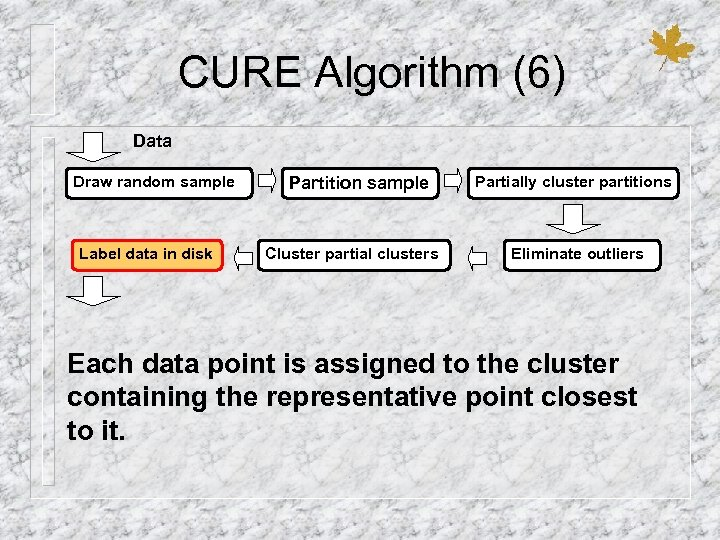CURE Algorithm (6) Data Draw random sample Label data in disk Partition sample Cluster partial clusters Partially cluster partitions Eliminate outliers Each data point is assigned to the cluster containing the representative point closest to it.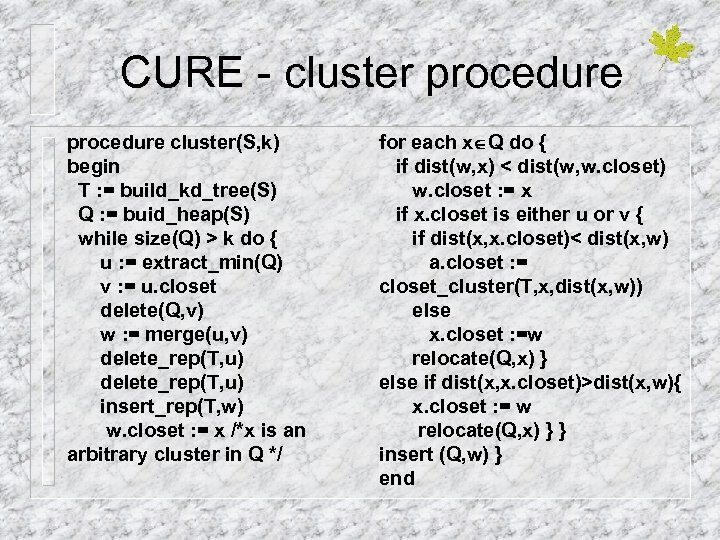CURE - cluster procedure cluster(S, k) begin T : = build_kd_tree(S) Q : = buid_heap(S) while size(Q) > k do { u : = extract_min(Q) v : = u. closet delete(Q, v) w : = merge(u, v) delete_rep(T, u) insert_rep(T, w) w. closet : = x /*x is an arbitrary cluster in Q */ for each x Q do { if dist(w, x) < dist(w, w. closet) w. closet : = x if x. closet is either u or v { if dist(x, x. closet)< dist(x, w) a. closet : = closet_cluster(T, x, dist(x, w)) else x. closet : =w relocate(Q, x) } else if dist(x, x. closet)>dist(x, w){ x. closet : = w relocate(Q, x) } } insert (Q, w) } end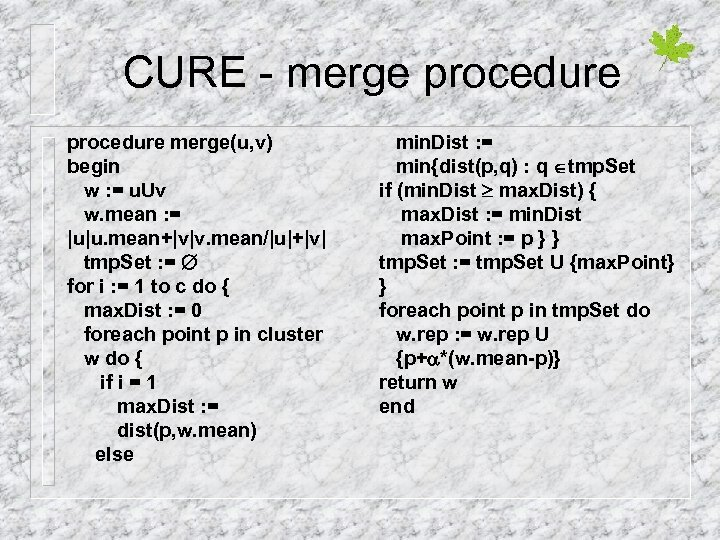CURE - merge procedure merge(u, v) begin w : = u. Uv w. mean : = |u|u. mean+|v|v. mean/|u|+|v| tmp. Set : = for i : = 1 to c do { max. Dist : = 0 foreach point p in cluster w do { if i = 1 max. Dist : = dist(p, w. mean) else min. Dist : = min{dist(p, q) : q tmp. Set if (min. Dist max. Dist) { max. Dist : = min. Dist max. Point : = p } } tmp. Set : = tmp. Set U {max. Point} } foreach point p in tmp. Set do w. rep : = w. rep U {p+ *(w. mean-p)} return w end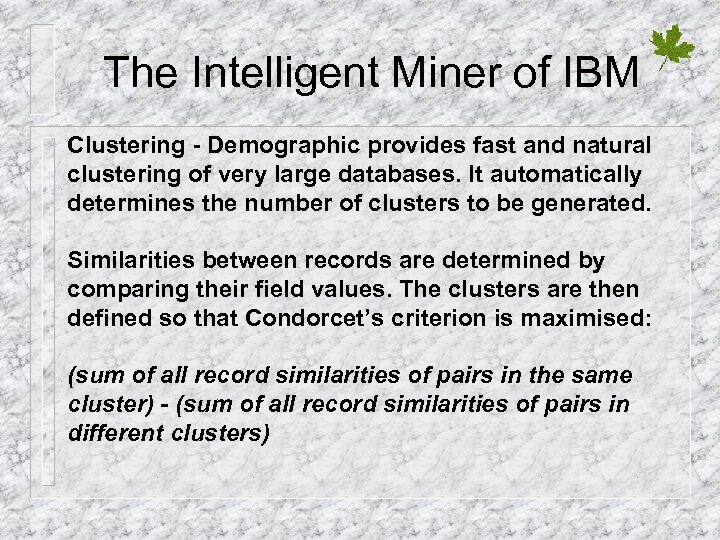The Intelligent Miner of IBM Clustering - Demographic provides fast and natural clustering of very large databases. It automatically determines the number of clusters to be generated. Similarities between records are determined by comparing their field values. The clusters are then defined so that Condorcet’s criterion is maximised: (sum of all record similarities of pairs in the same cluster) - (sum of all record similarities of pairs in different clusters)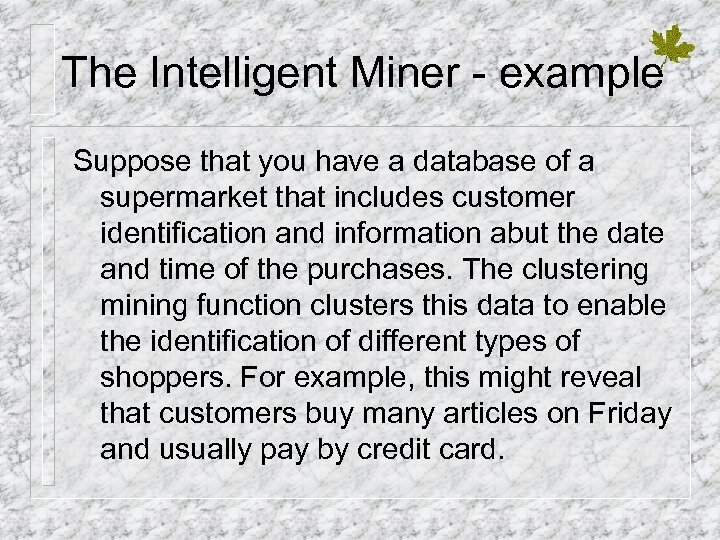The Intelligent Miner - example Suppose that you have a database of a supermarket that includes customer identification and information abut the date and time of the purchases. The clustering mining function clusters this data to enable the identification of different types of shoppers. For example, this might reveal that customers buy many articles on Friday and usually pay by credit card.